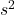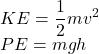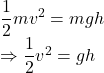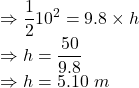Question

A ball thrown straight up into the air with a speed of 10m/s. If the ball has a mass of 0.3 Kg, how high does the ball go? Acceleration due to gravity is g=9.8 m/s2

1.Jezebel

5.10 m

Explanation:

Given that:

Initial velocity given to the ball, v = 10 m/s

Mass of the ball, m = 0.3 kg

Acceleration due to gravity, g = 9.8m/To find: The height upto which the ball will go =  ?

Solution:

Initially, the ball will have kinetic energy and at top point the velocity will be zero, it will have only potential energy due to height.

Formula for kinetic energy and potential energy:Applying conservation of energy principle:Putting the values of v and g to find h:The ball will go 5.10 m high.